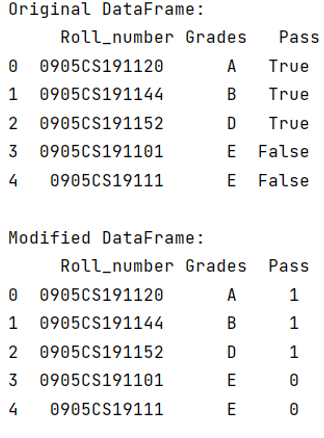# How to Map True/False to 1/0 in a Pandas DataFrame?

Given a Pandas DataFrame, we have to map True/False to 1/0. By Pranit Sharma Last updated : September 21, 2023

In programming, we sometimes use some specific values that an only have two values, either True or False. These values are known as Boolean values. These Boolean values have data type which has the same name i.e., Boolean. Also, Boolean values have their corresponding values with data type int also. 1 and 0 are the integer values which represent True and False respectively.

## Problem statement

In this article, we are going to convert all the Boolean values in the format of True/False into the format of 1/0.

## Mapping True/False to 1/0 in a Pandas DataFrame

For this purpose, we are going to use astype() method. It is used for casting the pandas object to a specified dtype, it can also be used for converting any suitable existing column to a categorical type.

Note

To work with pandas, we need to import pandas package first, below is the syntax:

```import pandas as pd
```

Let us understand with the help of an example.

## Python Program to Map True/False to 1/0 in a Pandas DataFrame

```# Importing pandas package
import pandas as pd

# Creating a dictionary
d = {
'Roll_number':['0905CS191120','0905CS191144','0905CS191152','0905CS191101','0905CS19111'],
'Pass':[True,True,True,False,False]
}

# Creating a DataFrame
df = pd.DataFrame(d)

# Display original DataFrame
print("Original DataFrame:\n",df,"\n")

# Converting Boolean values True/False into 1/0
df['Pass'] = df['Pass'].astype(int)

# Display modified DataFrame
print("Modified DataFrame:\n",df)
```

### Output

The output of the above program is: